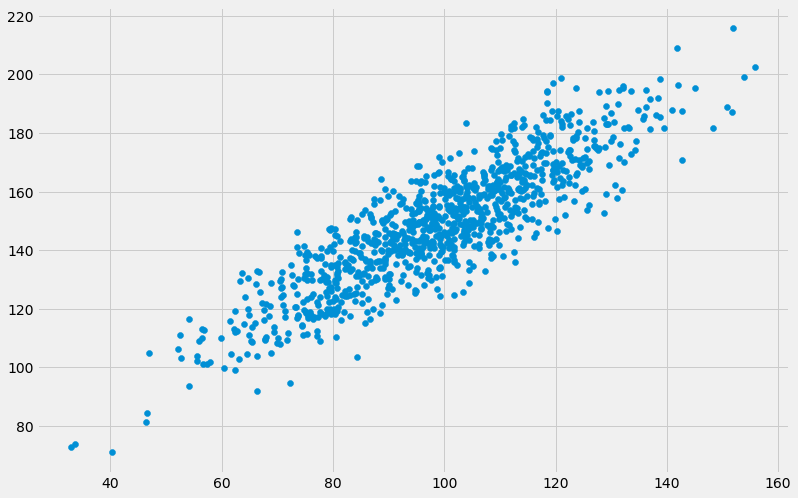# Applied Data Science Notebook in Python for Beginners to Professionals¶

## Data Science Project – A Guide to Calculate Correlation Between Variables for Machine Learning in Python¶

### Machine Learning for Beginners - A Guide to Calculate Correlation Between Variables for Machine Learning in Python¶

For more projects visit: https://setscholars.net

• There are 5000+ free end-to-end applied machine learning and data science projects available to download at SETSscholar. SETScholars is a Science, Engineering and Technology Scholars community.
In :
# Suppress warnings in Jupyter Notebooks
import warnings
warnings.filterwarnings("ignore")

import matplotlib.pyplot as plt
plt.style.use('fivethirtyeight')


In this notebook, we will learn how to Calculate Correlation Between Variables for Machine Learning in Python.

## Python Codes¶

### Create a simulated dataset¶

In :
# generate related variables
from numpy import mean
from numpy import std
from numpy.random import randn
from numpy.random import seed
from matplotlib import pyplot

# seed random number generator
seed(412)

# prepare data
data1 = 20 * randn(1000) + 100
data2 = data1 + (10 * randn(1000) + 50)

# summarize
print('data1: mean=%.3f stdv=%.3f' % (mean(data1), std(data1)))
print('data2: mean=%.3f stdv=%.3f' % (mean(data2), std(data2)))
print()

# plot
pyplot.figure(figsize=(12,8))
pyplot.scatter(data1, data2)
pyplot.show()

data1: mean=98.807 stdv=18.773
data2: mean=148.807 stdv=21.149## Calculate Correlation Between Variables for Machine Learning in Python¶

### Covariance¶

In :
# calculate the covariance between two variables
from numpy.random import randn
from numpy.random import seed
from numpy import cov

# seed random number generator
seed(412)

# prepare data
data1 = 20 * randn(1000) + 100
data2 = data1 + (10 * randn(1000) + 50)

# calculate covariance matrix
covariance = cov(data1, data2)
print(covariance)

[[352.78813306 348.56882819]
[348.56882819 447.74847497]]

In [ ]:



### Pearson’s Correlation¶

In :
# calculate the Pearson's correlation between two variables
from numpy.random import randn
from numpy.random import seed
from scipy.stats import pearsonr

# seed random number generator
seed(412)

# prepare data
data1 = 20 * randn(1000) + 100
data2 = data1 + (10 * randn(1000) + 50)

# calculate Pearson's correlation
corr, _ = pearsonr(data1, data2)
print('Pearsons correlation: %.3f' % corr)

Pearsons correlation: 0.877

In [ ]:



### Spearman’s Correlation¶

In :
# calculate the spearmans's correlation between two variables
from numpy.random import randn
from numpy.random import seed
from scipy.stats import spearmanr

# seed random number generator
seed(412)

# prepare data
data1 = 20 * randn(1000) + 100
data2 = data1 + (10 * randn(1000) + 50)

# calculate spearman's correlation
corr, _ = spearmanr(data1, data2)
print('Spearmans correlation: %.3f' % corr)

Spearmans correlation: 0.861

In [ ]:



## Summary¶

In this coding recipe, we discussed how to Calculate Correlation Between Variables for Machine Learning in Python.

Specifically, we have learned the followings:

• How to calculate Correlation Between Variables for Machine Learning in Python.
In [ ]: